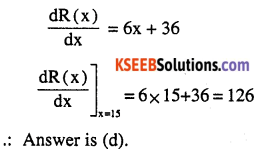# 2nd PUC Maths Question Bank Chapter 6 Application of Derivatives Ex 6.1

Students can Download Maths Chapter 6 Application of Derivatives Ex 6.1 Questions and Answers, Notes Pdf, 2nd PUC Maths Question Bank with Answers helps you to revise the complete Karnataka State Board Syllabus and score more marks in your examinations.

## Karnataka 2nd PUC Maths Question Bank Chapter 6 Application of Derivatives Ex 6.1

### 2nd PUC Maths Application of Derivatives NCERT Text Book Questions and Answers Ex 6.1

Question 1.
Find the rate of change of the area of a circle with respect to its radius r when
(a) r = 3 cm
(b) r = 4 cmQuestion 2.
The volume of a cube is increasing at the rate of 8 cm3/s. How fast is the surface area increasing when the length of an edge is 12 cm?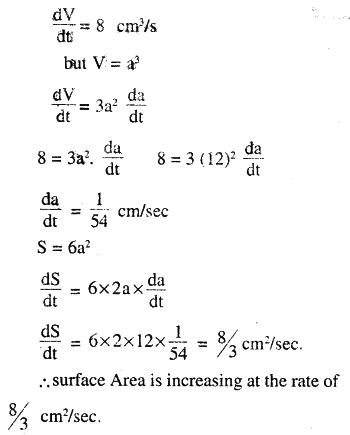Question 3.
The radius of a circle is increasing uniformly at the rate of 3 cm/s. Find the rate at which the area of the circle is increasing when the radius is 10 cm.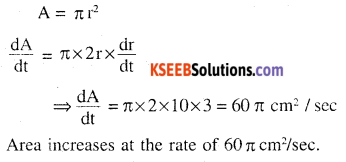Question 4.
An edge of a variable cube is increasing at the rate of 3 cm/s. How fast is the volume of the cube increasing when the edge is 10 cm long?Question 5.
A stone is dropped into a quiet lake and waves move in circles at the speed of 5 cm/s. At the instant when the radius of the circular wave is 8 cm, how fast is the enclosed area increasing?Question 6.
The radius of a circle is increasing at the rate of 0.7 cm/s. What is the rate of increase of its circumference?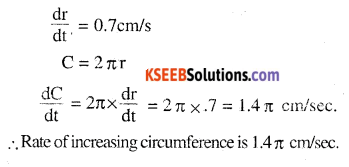Question 7.
The length x of a rectangle is decreasing at the rate of 5 cm/minute and the width y is increasing at the rate of 4 cm/minute. When x = 8cm and y = 6cm, find the rates of change of
(a) the perimeter, and
(b) the area of the rectangle.Question 8.
A balloon, which always remains spherical on inflation, is being inflated by pumping in 900 cubic centimetres of gas per second. Find the rate at which the radius of the balloon increases when the radius is 15 cm.Question 9.
A balloon, which always remains spherical has a variable radius. Find the rate at which its volume is increasing with the radius when the latter is 10 cm.Question 10.
A ladder 5 in long is leaning against a wall. The bottom of the ladder is pulled along the ground, away from the wall, at the rate of 2cm/s. How fast is its height on the wall decreasing when the foot of the ladder is 4 m away from the wall ?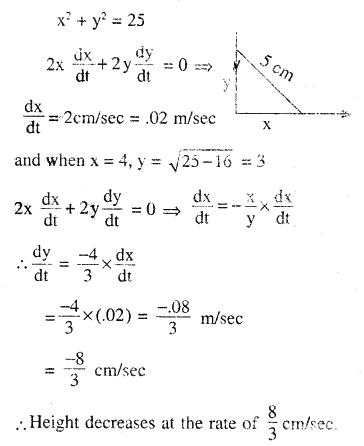Question 11.
Find the points on the curve at which the y-coordinate is changing 8 times as fast as the x-coordinate.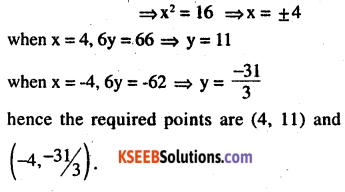Question 12.
The radius of an air bubble is increasing at the rate of 1/2 cm/s. At what rate is the volume of the bubble increasing when the radius is 1 cm?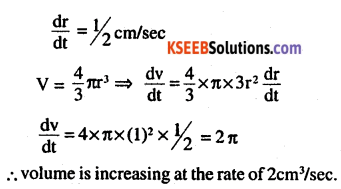Question 13.
A balloon, which always remains spherical, has a variable diameter 3/2 (2x + 1). Find the rate of change of its volume with respect to x.Question 14.
Sand is pouring from a pipe at the rate of 12 cm3/s. The falling sand forms a cone on the ground in such a way that the height of the cone is always one-sixth of the radius of the base. How fast is the height of the sand cone increasing when the height is 4 cm?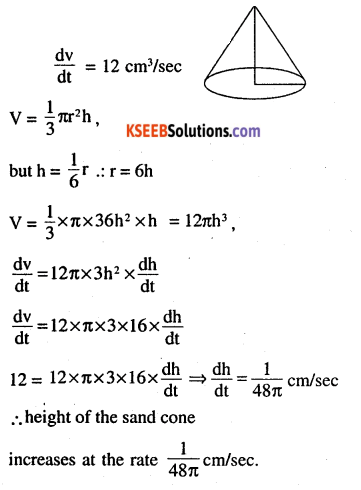Question 15.
The total cost C(x) in Rupees associated with the production of x units of an item is given by C(x) = 0.007x3 – 0.003x2 + 15x + 4000. Find the marginal cost when 17 units are produced.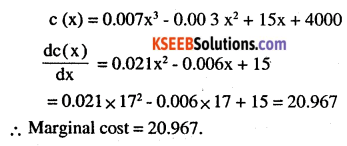Question 16.
The total revenue in Rupees received from the sale of x units of a product is given by R(x) = 13x2 + 26x + 15. Find the marginal revenue when x = 7. Choose the correct answer in the Exercises 17 and 18.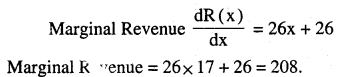Question 17.
The rate of change of the area of a circle with respect to its radius r at r = 6 cm is
(A) 10π
(B) 12π
(C) 8π
(D) 11π